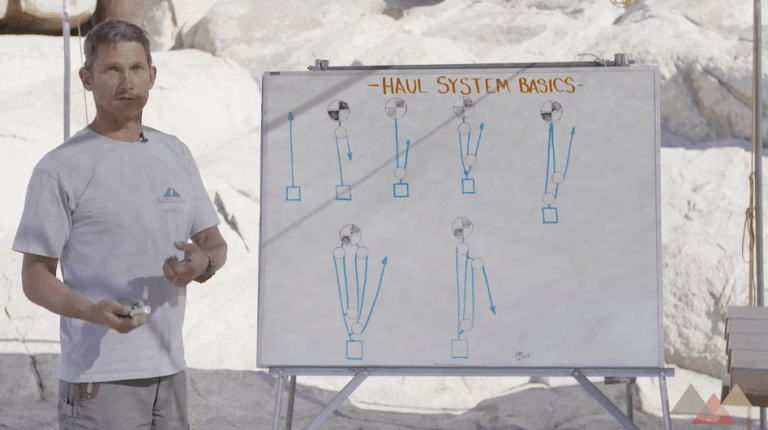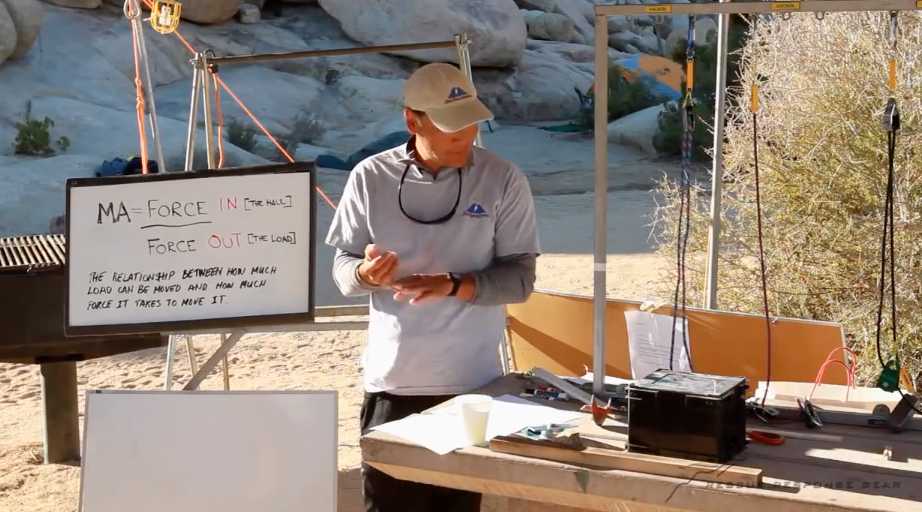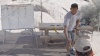## Written By: Peak Rescue Institute`From Rigging Lab Academy Course Mechanical Advantage Theory`

There are three categories of mechanical advantages using pulleys: SimpleCompound, and Complex.

1. Simple MA: A Simple MA consists of a pulley system that has a single haul connection between the load and the haul team.
2. Compound MA: A Compound MA is a simple mechanical advantage system pulling on the haul line of another simple mechanical advantage. Multiplying the two systems will give the total advantage.
3. Complex MA (see more below)### 5 Rules to Help Determine Simple & Compound Mechanical Advantage Systems:

There are five rules that can be used to determine simple and compound mechanical advantage systems.

1. Rule #1: If the pulley closest to the haulers is on the anchor, the pulley is only considered a change of direction (cd). The same rule applies to ANY pulley system.
2. Rule #2: If the rope used in the pulley system is tied to the anchor, the ideal mechanical advantage (IMA) will be EVEN (i.e., 2:1, 4:1. 6:1, etc.)
3. Rule #3: If the rope used in the pulley system is tied to the load, the ideal mechanical advantage (IMA) will be ODD (i.e., 1:1, 3:1. 5:1, etc.)
4. Rule #4: To determine the IMA of a simple pulley system, count the ropes between the anchor and the load. Do not count the ropes between two anchors.
5. Rule #5: A simple MA pulling on the haul line of another simple MA is called a compound MA system.

### What is a Complex MA?

A Complex MA system is neither simple or compound, and the above rules will not work in determining the system. The only way in determining the mechanical advantage of a complex MA system is by calculating the “tension units”. See “Critical Thinking On Mechanical Advantage Systems” at the end of this section for further details.

The combinations of pulleys that can be incorporated in an MA system are infinite. With this in mind, how many pulleys are needed, and what are the characteristics of a quality haul system?Hidden Content

Lance

## Free eBook

###### Learn how to master 10 major anchor systems with The Foundations of Rescue & Rigging Anchor Systems eBook.•SEO Referral Program says: